#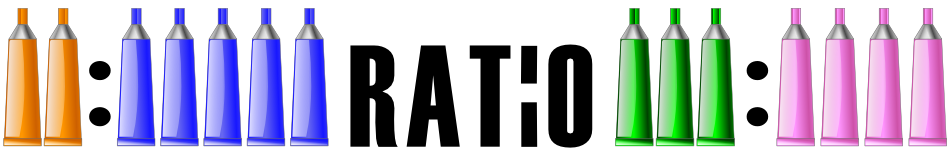There are 366 different Starters of The Day, many to choose from. You will find below some starters on the topic of Ratio. A lesson starter does not have to be on the same topic as the main part of the lesson or the topic of the previous lesson. It is often very useful to revise or explore other concepts by using a starter based on a totally different area of Mathematics.

Main Page

### Ratio Starters:A real life situation requiring the division an amount of money according to a given ratio.If six girls can plant 90 trees in a day. How many trees can ten girls plant in a day? The unitary method.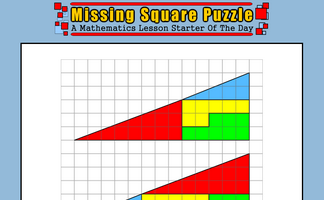The missing square puzzle is an optical illusion used to help students reason about geometrical figures.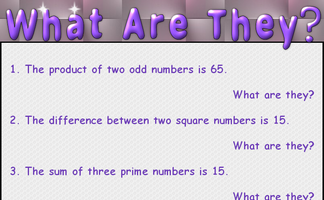A starter about sums, products, differences, ratios, square and prime numbers.

## Exercises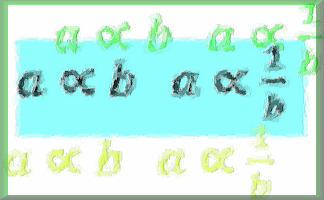#### Direct and Inverse Proportion

A self-marking exercise in solving direct and inverse variation problems.#### Dividing in a Ratio

A slide presentation showing how to divide a quantity in a given ratio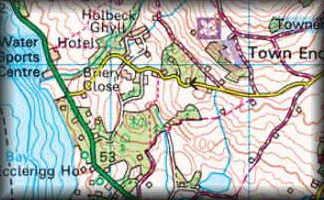#### Map Scales

Test your understanding of map scales expressed as ratios with this self marking quiz.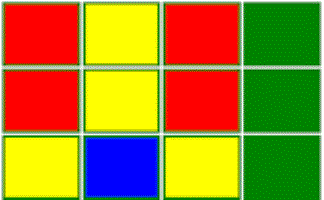#### Pattern Clues

An interactive activity challenging you to reproduce a pattern of coloured squares according to given clues.#### Ratio

A self marking exercise on using ratio notation, reducing a ratio to its simplest form and dividing a given quantity into a number of parts in proportion to a given ratio.#### Ratio Clues

Arrange the ratio clues in the clouds in a logical order to work out the values of the twelve letters.#### Recipe Ratios

Learn the mathematics required to adapt recipes to serve a different number of people.#### Scale Drawings

Measure line segments and angles in geometric figures, including interpreting scale drawings.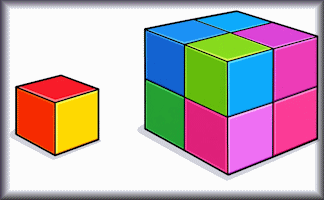#### Similar Shapes

Questions about the scale factors of lengths, areas and volumes of similar shapes.#### Unit Pricing

Calculate the unit cost of items to earn jigsaw pieces that make a joke.#### Unitary Method

Ten questions which can be solved using the unitary method.

### Search

The activity you are looking for may have been classified in a different way from the way you were expecting. You can search the whole of Transum Maths by using the box below.

Have today's Starter of the Day as your default homepage. Copy the URL below then select
Tools > Internet Options (Internet Explorer) then paste the URL into the homepage field.

Set as your homepage (if you are using Internet Explorer)

Do you have any comments? It is always useful to receive feedback and helps make this free resource even more useful for those learning Mathematics anywhere in the world. Click here to enter your comments.For All: